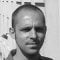Get access to premium HV/MV/LV technical articles, electrical engineering guides and research studies. Check new membership plans!

# 4 example calculations of compensation for reactive power

Home / Technical Articles / 4 example calculations of compensation for reactive power

## Reactive power

First, let’s say some words about basics of the reactive power in system. Reactive current arises in every electrical system.

Not only large loads, but smaller loads as well require reactive power. Generators and motors produce reactive power, which causes unnecessary burdens to and power losses in the lines.

Reactive power is necessary to generate magnetic fields, e.g. in motors, transformers and generators. This power oscillates between the source and the load and represents an additional loading.

Power supply companies and the consumers of this electrical energy are interested in reducing these disadvantages as well as possible. On the other hand, non-linear loads and phase-controlled inverters cause harmonics, which lead to voltage changes and a decrease in the power factor. In order to reduce these harmonics, series resonant (filter) circuits are used.

Now, let’s take few examples to calculate the following:

### Example 1 – Determination of Capacitive Power

A load has an effective power of P = 50 kW at 400 V and the power factor is to be compensated from cosφ = 0.75 to cosφ = 0.95. Determine the required capacitive power. The power and current before compensation are:The power and current after compensation are:The required capacitive power  is:Go back to calculations ↑

### Example 2 – Capacitive Power With k Factor

The capacitive power can be determined with the factor k for a given effective power. The k factor is read from a table 1 – Multipliers to determine capacitor kilovars required for power factor correction (see below) and multiplied by the effective power. The result is the required capacitive power.

For an increase in the power factor from cosφ = 0.75 to cosφ = 0.95, from the table 1 we find a factor k = 0.55:Go back to calculations ↑

### Example 3 – Determination of Cable Cross-Section

A three-phase power of 250 kW, with Un = 400 V, at 50 Hz is to be transmitted over a cable 80 m in length. The voltage drop must not exceed 4% =16 V. The power factor is to be increased from cosφ = 0.7 to cosφ = 0.95. What is the required cable cross-section?The current consumption before compensation is:The current consumption after compensation is:The effective resistance per unit length for 516 A is:According to Table 2 (see below) we must choose a cable with a cross-section of 4 × 95 mm2. The effective resistance per unit length for 380 A is:Here, a cable cross-section of 4 × 70 mm2 is required. As this example illustrates, the improved power factor leads to lower costs because of the reduced cross-section.

Go back to calculations ↑

### Example 4 – Calculation of the c/k Value

Given a 150 condenser battery, i.e. 5 stages of 30 each, a supply voltage of 400 V, and an instrument transformer with a k of 500 A/5 A, how large is the c/k value? The ratio c/k is given by.Go back to calculations ↑

### Tables

Table 1 – Multipliers to Determine Capacitor Kilovars Required for Power Factor Correction

Table 2 – Resistance per unit length for (Cu) cable with plastic insulation

Go back to calculations ↑

References

1. Analysis and design of low voltage power systems by Ismail Kasikci (Purchase hardcover from Amazon)
2. Power factor correction – A guide for the plant engineer by EATON

Get access to premium HV/MV/LV technical articles, electrical engineering guides, research studies and much more! It helps you to shape up your technical skills in your everyday life as an electrical engineer.### Edvard Csanyi

Electrical engineer, programmer and founder of EEP. Highly specialized for design of LV/MV switchgears and LV high power busbar trunking (<6300A) in power substations, commercial buildings and industry facilities. Professional in AutoCAD programming.

1.Thanks
Aug 19, 2019

Thanks

2.Yann saidi
Aug 19, 2019

Thank you a lot

3.andda
Apr 10, 2019

How do we know how many and what kvar load is needed to compensate reactive power .Is there any table ?

4.ARNEL CORPUZ
Jan 08, 2018

thank you for this. this is very helpful.

•Edvard
Jan 08, 2018

Thank you Arnel!

5.Tomáš
Feb 17, 2017

Hey, I do not think that the pic b is correct. There are strange angles at Ur.
cos phi = 1 means 0 deg between current and voltage so it is not capacitave nor reactive load but pure resistive.

6.Abass
Jan 24, 2017

Many thanks Edvard.

7.Josè Severino Queiroz
Jan 23, 2017

This is a good and practical method to get better the performance of the system mainly the losses and voltage regulations. Thank you..

8.Aleksandar Kovac
Jan 23, 2017

very good.
thanks

9.Amine
Jan 20, 2017

very nice thank you

10.gamal
Jan 20, 2017

Thank for usefull report

### Subscribe to Weekly Digest

Get email alert whenever we publish new electrical guides and articles.